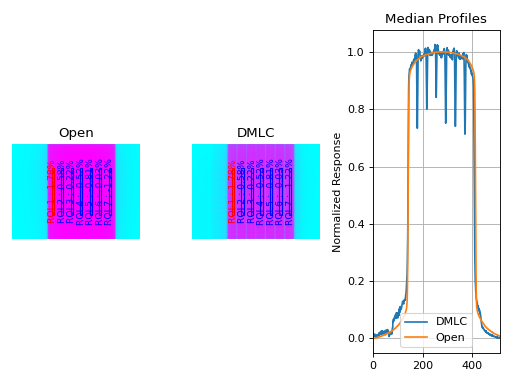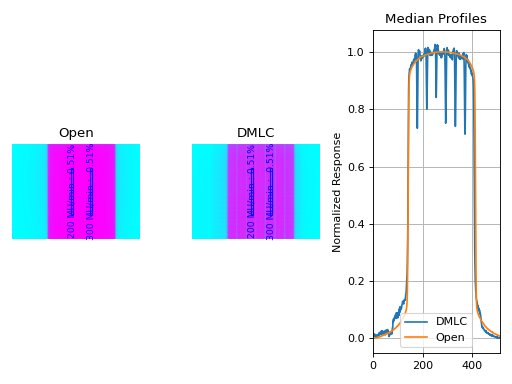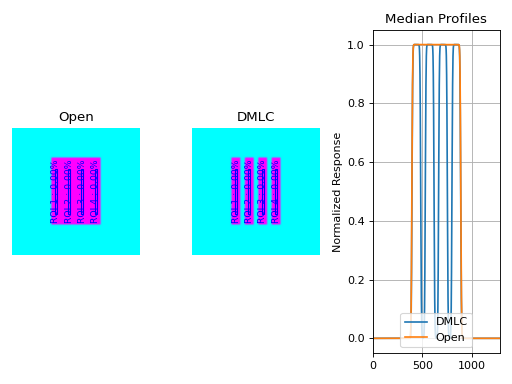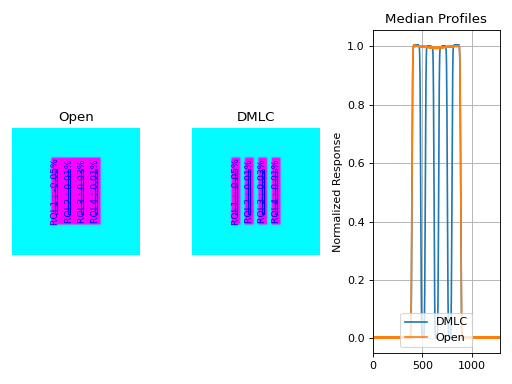# VMAT¶

## Overview¶

The VMAT module consists of the class VMAT, which is capable of loading an EPID DICOM Open field image and MLC field image and analyzing the images according to the Varian RapidArc QA tests and procedures, specifically the Dose-Rate & Gantry-Speed (DRGS) and Dose-Rate & MLC speed (DRMLC) tests.

Features:

• Do both tests - Pylinac can handle either DRGS or DRMLC tests.
• Automatic offset correction - Older VMAT tests had the ROIs offset, newer ones are centered. No worries, pylinac finds the ROIs automatically.
• Automatic open/DMLC identification - Pass in both images–don’t worry about naming. Pylinac will automatically identify the right images.

Note

There are two classes in the VMAT module: DRGS and DRMLC. Each have the exact same methods. Anytime one class is used here as an example, the other class can be used the same way.

## Running the Demos¶

For this example we will use the DRGS class:

from pylinac import DRGS
DRGS.run_demo()Results will be printed to the console and a figure showing both the Open field and MLC field image will pop up:

Dose Rate & Gantry Speed
Test Results (Tol. +/-1.5%): PASS
Max Deviation: 1.01%
Absolute Mean Deviation: 0.459%


## Image Acquisition¶

If you want to perform these specific QA tests, you’ll need DICOM plan files that control the linac precisely to deliver the test fields. These can be downloaded from my.varian.com. Once logged in, search for RapidArc and you should see two items called “RapidArc QA Test Procedures and Files for TrueBeam”; there will be a corresponding one for C-Series. Use the RT Plan files and follow the instructions, not including the assessment procedure, which is the point of this module. Save & move the VMAT images to a place you can use pylinac.

## Typical Use¶

The VMAT QA analysis follows what is specified in the Varian RapidArc QA tests and assumes your tests will run the exact same way. Import the appropriate class:

from pylinac import DRGS, DRMLC


The minimum needed to get going is to:

• Load images – Loading the EPID DICOM images into your VMAT class object can be done by passing the file paths, passing a ZIP archive, or passing a URL:

open_img = "C:/QA Folder/VMAT/open_field.dcm"
dmlc_img = "C:/QA Folder/VMAT/dmlc_field.dcm"
mydrgs = DRGS(image_paths=(open_img, dmlc_img))  # use the DRMLC class the exact same way

# from zip
mydrmlc = DRMLC.from_zip(r'C:/path/to/zip.zip')

# from a URL
mydrgs = DRGS.from_url('http://myserver.org/vmat.zip')


Finally, if you don’t have any images, you can use the demo ones provided:

mydrgs = DRGS.from_demo_images()
mydrmlc = DRMLC.from_demo_images()

• Analyze the images – Once the images are loaded, tell the class to analyze the images. See the Algorithm section for details on how this is done. Tolerance can also be passed and has a default value of 1.5%:

mydrgs.analyze(tolerance=1.5)

• View/Save the results – The VMAT module can print out the summary of results to the console as well as draw a matplotlib image to show where the segments were placed and their values:

# print results to the console
print(mydrgs.results())
# view analyzed images
mydrgs.plot_analyzed_image()PDF reports can also be generated:

myvmat.publish_pdf('drgs.pdf')


## Customizing the analysis¶

You can alter both the segment size and segment positions as desired.

To change the segment size:

drgs = DRGS.from_demo_image()
drgs.analyze(..., segment_size_mm=(10, 150))  # ROI segments will now be 10mm wide by 150mm tall
# same story for DRMLC


To change the x-positions of the ROI segments, the class property must be changed. This is different behavior because by default the x-positions are different for the DRGS and DRMLC test:

from pylinac import DRGS, DRMLC

DRGS.SEGMENT_X_POSITIONS_MM = (-100, -80, ...)
# proceed as normal
my_drgs = DRGS(...)

# DRMLC must be addressed separately
DRMLC.SEGMENT_X_POSITIONS_MM = (-40, -10, 10, 40)
my_drmlc = DRMLC(...)


## Accessing Data¶

Changed in version 3.0.

Using the VMAT module in your own scripts? While the analysis results can be printed out, if you intend on using them elsewhere (e.g. in an API), they can be accessed the easiest by using the results_data() method which returns a VMATResult instance.

Note

While the pylinac tooling may change under the hood, this object should remain largely the same and/or expand. Thus, using this is more stable than accessing attrs directly.

Continuing from above:

data = my_drmlc.results_data()
data.test_type
data.passed
# and more

# return as a dict
data_dict = my_drmlc.results_data(as_dict=True)
data_dict['test_type']
...


## Algorithm¶

The VMAT analysis algorithm is based on the Varian RapidArc QA Test Procedures for C-Series and Truebeam. Two tests (besides Picket Fence, which has its own module) are specified. Each test takes 10x0.5cm samples, each corresponding to a distinct section of radiation. A corrected reading of each segment is made, defined as: $$M_{corr}(x) = \frac{M_{DRGS}(x)}{M_{open}(x)} * 100$$. The reading deviation of each segment is calculated as: $$M_{deviation}(x) = \frac{M_{corr}(x)}{M_{corr}} * 100 - 100$$, where $$M_{corr}$$ is the average of all segments.

The algorithm works like such:

Allowances

• The images can be acquired at any SID.
• The images can be acquired with any EPID (aS500, aS1000, aS1200).

Restrictions

Warning

Analysis can fail or give unreliable results if any Restriction is violated.

• The tests must be delivered using the DICOM RT plan files provided by Varian which follow the test layout of Ling et al.
• The images must be acquired with the EPID.

Pre-Analysis

• Determine image scaling – Segment determination is based on offsets from the center pixel of the image. However, some physicists use 150 cm SID and others use 100 cm, and others can use a clinical setting that may be different than either of those. To account for this, the SID is determined and then scaling factors are determined to be able to perform properly-sized segment analysis.

Analysis

Note

Calculations tend to be lazy, computed only on demand. This represents a nominal analysis where all calculations are performed.

• Calculate sample boundaries – Segment positions are always the same within the image. The x-positions are based on the FWHM of the detected field. This allows for old and new style tests that have an x-offset from each other. These values are then scaled with the image scaling factor determined above.
• Calculate the corrected reading – For each segment, the mean pixel value is determined for both the open and DMLC image. These values are used to determine the corrected reading: $$M_{corr}$$.
• Calculate sample and segment ratios – The sample values of the DMLC field are divided by their corresponding open field values.
• Calculate segment deviations – Segment deviation is then calculated once all the corrected readings are determined. The average absolute deviation is also calculated.

Post-Analysis

• Test if segments pass tolerance – Each segment is checked to see if it was within the specified tolerance. If any samples fail, the whole test is considered failing.

## Benchmarking the Algorithm¶

With the image generator module we can create test images to test the VMAT algorithm on known results. This is useful to isolate what is or isn’t working if the algorithm doesn’t work on a given image and when commissioning pylinac.

Note

The below examples are for the DRMLC test but can equally be applied to the DRGS tests as well.

### Perfect Fields¶

In this example, we generate a perfectly flat set of images.

The script will generate the files, but you can also download them here: perfect_open_drmlc.dcm perfect_dmlc_drmlc.dcm.

import pylinac
from pylinac.core.image_generator import GaussianFilterLayer, PerfectFieldLayer, AS1200Image

# open image
open_path = 'perfect_open_drmlc.dcm'
as1200 = AS1200Image()
as1200.generate_dicom(file_out_name=open_path)

# DMLC image
dmlc_path = 'perfect_dmlc_drmlc.dcm'
as1200 = AS1200Image()
for offset in (-40, -10, 20, 50):
as1200.generate_dicom(file_out_name=dmlc_path)

# analyze it
vmat = pylinac.DRMLC(image_paths=(open_path, dmlc_path))
vmat.analyze()
print(vmat.results())
vmat.plot_analyzed_image()with output:

Dose Rate & MLC Speed
Test Results (Tol. +/-1.5%): PASS
Max Deviation: 0.0%
Absolute Mean Deviation: 0.0%


### Noisy, Realistic¶

We now add a horn effect and random noise to the data:

import pylinac
from pylinac.core.image_generator import GaussianFilterLayer, FilteredFieldLayer, AS1200Image, RandomNoiseLayer

# open image
open_path = 'noisy_open_drmlc.dcm'
as1200 = AS1200Image()
as1200.generate_dicom(file_out_name=open_path)

# DMLC image
dmlc_path = 'noisy_dmlc_drmlc.dcm'
as1200 = AS1200Image()
for offset in (-40, -10, 20, 50):
as1200.generate_dicom(file_out_name=dmlc_path)

# analyze it
vmat = pylinac.DRMLC(image_paths=(open_path, dmlc_path))
vmat.analyze()
print(vmat.results())
vmat.plot_analyzed_image()with output:

Dose Rate & MLC Speed
Test Results (Tol. +/-1.5%): PASS
Max Deviation: 0.0332%
Absolute Mean Deviation: 0.0257%


### Erroneous data¶

Let’s now get devious and randomly adjust the height of each ROI (effectively changing the apparent MLC speed):

Note

Due to the purposely random nature shown below, this exact result is likely not reproducible, nor was it intended to be. To get reproducible behavior, use numpy with a seed value.

import random

import pylinac
from pylinac.core.image_generator import GaussianFilterLayer, FilteredFieldLayer, AS1200Image, RandomNoiseLayer

# open image
open_path = 'noisy_open_drmlc.dcm'
as1200 = AS1200Image()
as1200.generate_dicom(file_out_name=open_path)

# DMLC image
dmlc_path = 'noisy_dmlc_drmlc.dcm'
as1200 = AS1200Image()
for offset in (-40, -10, 20, 50):
as1200.add_layer(FilteredFieldLayer((150, 20), cax_offset_mm=(0, offset), alpha=random.uniform(0.93, 1)))
as1200.generate_dicom(file_out_name=dmlc_path)

# analyze it
vmat = pylinac.DRMLC(image_paths=(open_path, dmlc_path))
vmat.analyze()
print(vmat.results())
vmat.plot_analyzed_image()with an output of:

Dose Rate & MLC Speed
Test Results (Tol. +/-1.5%): FAIL
Max Deviation: 2.12%
Absolute Mean Deviation: 1.13%


## API Documentation¶

### Main classes¶

These are the classes a typical user may interface with.

class pylinac.vmat.DRGS(image_paths: Sequence[Union[str, BinaryIO]])[source]

Class representing a Dose-Rate, Gantry-speed VMAT test. Will accept, analyze, and return the results.

Parameters: image_paths (iterable (list, tuple, etc)) – A sequence of paths to the image files.
static run_demo()[source]

Run the demo for the Dose Rate & Gantry Speed test.

analyze(tolerance: Union[float, int] = 1.5, segment_size_mm: Tuple = (5, 100))

Analyze the open and DMLC field VMAT images, according to 1 of 2 possible tests.

Parameters: tolerance (float, int, optional) – The tolerance of the sample deviations in percent. Default is 1.5. Must be between 0 and 8. segment_size_mm (tuple(int, int)) – The (width, height) of the ROI segments in mm.
avg_abs_r_deviation

Return the average of the absolute R_deviation values.

avg_r_deviation

Return the average of the R_deviation values, including the sign.

classmethod from_demo_images()

Construct a VMAT instance using the demo images.

classmethod from_url(url: str)

Load a ZIP archive from a URL. Must follow the naming convention.

Parameters: url (str) – Must point to a valid URL that is a ZIP archive of two VMAT images.
classmethod from_zip(path: str)

Load VMAT images from a ZIP file that contains both images. Must follow the naming convention.

Parameters: path (str) – Path to the ZIP archive which holds the VMAT image files.
max_r_deviation

Return the value of the maximum R_deviation segment.

plot_analyzed_image(show: bool = True)

Plot the analyzed images. Shows the open and dmlc images with the segments drawn; also plots the median profiles of the two images for visual comparison.

Parameters: show (bool) – Whether to actually show the image.
publish_pdf(filename: str, notes: str = None, open_file: bool = False, metadata: Optional[dict] = None)

Publish (print) a PDF containing the analysis, images, and quantitative results.

Parameters: filename ((str, file-like object}) – The file to write the results to. notes (str, list of strings) – Text; if str, prints single line. If list of strings, each list item is printed on its own line. open_file (bool) – Whether to open the file using the default program after creation. metadata (dict) – Extra data to be passed and shown in the PDF. The key and value will be shown with a colon. E.g. passing {‘Author’: ‘James’, ‘Unit’: ‘TrueBeam’} would result in text in the PDF like: ————– Author: James Unit: TrueBeam ————–
r_devs

Return the deviations of all segments as an array.

results() → str

A string of the summary of the analysis results.

Returns: The results string showing the overall result and deviation statistics by segment. str
results_data(as_dict=False) → Union[pylinac.vmat.VMATResult, dict]

Present the results data and metadata as a dataclass or dict. The default return type is a dataclass.

class pylinac.vmat.DRMLC(image_paths: Sequence[Union[str, BinaryIO]])[source]

Class representing a Dose-Rate, MLC speed VMAT test. Will accept, analyze, and return the results.

Parameters: image_paths (iterable (list, tuple, etc)) – A sequence of paths to the image files.
static run_demo()[source]

Run the demo for the MLC leaf speed test.

analyze(tolerance: Union[float, int] = 1.5, segment_size_mm: Tuple = (5, 100))

Analyze the open and DMLC field VMAT images, according to 1 of 2 possible tests.

Parameters: tolerance (float, int, optional) – The tolerance of the sample deviations in percent. Default is 1.5. Must be between 0 and 8. segment_size_mm (tuple(int, int)) – The (width, height) of the ROI segments in mm.
avg_abs_r_deviation

Return the average of the absolute R_deviation values.

avg_r_deviation

Return the average of the R_deviation values, including the sign.

classmethod from_demo_images()

Construct a VMAT instance using the demo images.

classmethod from_url(url: str)

Load a ZIP archive from a URL. Must follow the naming convention.

Parameters: url (str) – Must point to a valid URL that is a ZIP archive of two VMAT images.
classmethod from_zip(path: str)

Load VMAT images from a ZIP file that contains both images. Must follow the naming convention.

Parameters: path (str) – Path to the ZIP archive which holds the VMAT image files.
max_r_deviation

Return the value of the maximum R_deviation segment.

plot_analyzed_image(show: bool = True)

Plot the analyzed images. Shows the open and dmlc images with the segments drawn; also plots the median profiles of the two images for visual comparison.

Parameters: show (bool) – Whether to actually show the image.
publish_pdf(filename: str, notes: str = None, open_file: bool = False, metadata: Optional[dict] = None)

Publish (print) a PDF containing the analysis, images, and quantitative results.

Parameters: filename ((str, file-like object}) – The file to write the results to. notes (str, list of strings) – Text; if str, prints single line. If list of strings, each list item is printed on its own line. open_file (bool) – Whether to open the file using the default program after creation. metadata (dict) – Extra data to be passed and shown in the PDF. The key and value will be shown with a colon. E.g. passing {‘Author’: ‘James’, ‘Unit’: ‘TrueBeam’} would result in text in the PDF like: ————– Author: James Unit: TrueBeam ————–
r_devs

Return the deviations of all segments as an array.

results() → str

A string of the summary of the analysis results.

Returns: The results string showing the overall result and deviation statistics by segment. str
results_data(as_dict=False) → Union[pylinac.vmat.VMATResult, dict]

Present the results data and metadata as a dataclass or dict. The default return type is a dataclass.

class pylinac.vmat.VMATResult(test_type: str, tolerance_percent: float, max_deviation_percent: float, abs_mean_deviation: float, passed: bool, segment_data: Iterable[pylinac.vmat.SegmentResult])[source]

This class should not be called directly. It is returned by the results_data() method. It is a dataclass under the hood and thus comes with all the dunder magic.

Use the following attributes as normal class attributes.

test_type = None
tolerance_percent = None
max_deviation_percent = None
abs_mean_deviation = None
passed = None
segment_data = None
class pylinac.vmat.SegmentResult(passed: bool, x_position_mm: float, r_corr: float, r_dev: float, center_x_y: float)[source]

Bases: object

An individual segment/ROI result

passed = None
x_position_mm = None
r_corr = None
r_dev = None
center_x_y = None

### Supporting Classes¶

You generally won’t have to interface with these unless you’re doing advanced behavior.

class pylinac.vmat.VMATBase(image_paths: Sequence[Union[str, BinaryIO]])[source]

Bases: object

Parameters: image_paths (iterable (list, tuple, etc)) – A sequence of paths to the image files.
classmethod from_url(url: str)[source]

Load a ZIP archive from a URL. Must follow the naming convention.

Parameters: url (str) – Must point to a valid URL that is a ZIP archive of two VMAT images.
classmethod from_zip(path: str)[source]

Load VMAT images from a ZIP file that contains both images. Must follow the naming convention.

Parameters: path (str) – Path to the ZIP archive which holds the VMAT image files.
classmethod from_demo_images()[source]

Construct a VMAT instance using the demo images.

analyze(tolerance: Union[float, int] = 1.5, segment_size_mm: Tuple = (5, 100))[source]

Analyze the open and DMLC field VMAT images, according to 1 of 2 possible tests.

Parameters: tolerance (float, int, optional) – The tolerance of the sample deviations in percent. Default is 1.5. Must be between 0 and 8. segment_size_mm (tuple(int, int)) – The (width, height) of the ROI segments in mm.
results() → str[source]

A string of the summary of the analysis results.

Returns: The results string showing the overall result and deviation statistics by segment. str
results_data(as_dict=False) → Union[pylinac.vmat.VMATResult, dict][source]

Present the results data and metadata as a dataclass or dict. The default return type is a dataclass.

r_devs

Return the deviations of all segments as an array.

avg_abs_r_deviation

Return the average of the absolute R_deviation values.

avg_r_deviation

Return the average of the R_deviation values, including the sign.

max_r_deviation

Return the value of the maximum R_deviation segment.

plot_analyzed_image(show: bool = True)[source]

Plot the analyzed images. Shows the open and dmlc images with the segments drawn; also plots the median profiles of the two images for visual comparison.

Parameters: show (bool) – Whether to actually show the image.
publish_pdf(filename: str, notes: str = None, open_file: bool = False, metadata: Optional[dict] = None)[source]

Publish (print) a PDF containing the analysis, images, and quantitative results.

Parameters: filename ((str, file-like object}) – The file to write the results to. notes (str, list of strings) – Text; if str, prints single line. If list of strings, each list item is printed on its own line. open_file (bool) – Whether to open the file using the default program after creation. metadata (dict) – Extra data to be passed and shown in the PDF. The key and value will be shown with a colon. E.g. passing {‘Author’: ‘James’, ‘Unit’: ‘TrueBeam’} would result in text in the PDF like: ————– Author: James Unit: TrueBeam ————–
class pylinac.vmat.Segment(center_point: pylinac.core.geometry.Point, open_image: pylinac.core.image.DicomImage, dmlc_image: pylinac.core.image.DicomImage, tolerance: Union[float, int])[source]

A class for holding and analyzing segment data of VMAT tests.

For VMAT tests, there are either 4 or 7 ‘segments’, which represents a section of the image that received radiation under the same conditions.

r_dev

The reading deviation (R_dev) from the average readings of all the segments. See RTD for equation info.

Type: float
r_corr

The corrected reading (R_corr) of the pixel values. See RTD for explanation and equation info.

Type: float
passed

Specifies where the segment reading deviation was under tolerance.

Type: boolean
r_corr

Return the ratio of the mean pixel values of DMLC/OPEN images.

bl_corner

The location of the bottom left corner.

br_corner

The location of the bottom right corner.

passed

Return whether the segment passed or failed.

plot2axes(axes: matplotlib.axes._axes.Axes, edgecolor: str = 'black', angle: float = 0.0, fill: bool = False, alpha: float = 1, facecolor: str = 'g', label=None)

Plot the Rectangle to the axes.

Parameters: axes (matplotlib.axes.Axes) – An MPL axes to plot to. edgecolor (str) – The color of the circle. angle (float) – Angle of the rectangle. fill (bool) – Whether to fill the rectangle with color or leave hollow.
tl_corner

The location of the top left corner.

tr_corner

The location of the top right corner.

get_bg_color() → str[source]

Get the background color of the segment when plotted, based on the pass/fail status.